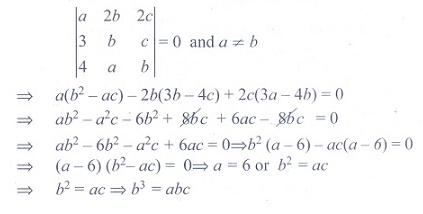# Chapter 7: Matrices and Determinants - Online Test

Q1. The value of x, for which the matrix A =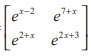is singular is
Explaination / Solution: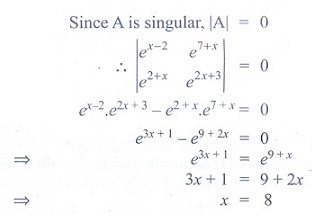Q2. If the points (x, −2), (5, 2), (8,8) are collinear, then x is equal to
Explaination / Solution: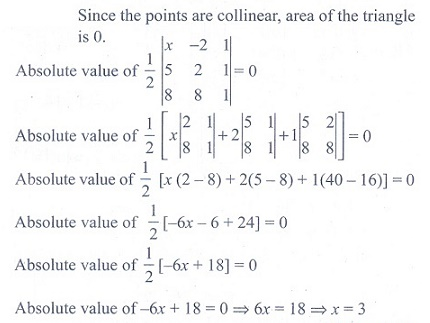Q3. If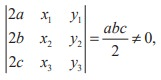= abc/2 ≠ 0, then the area of the triangle whose vertices are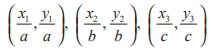is
Explaination / Solution: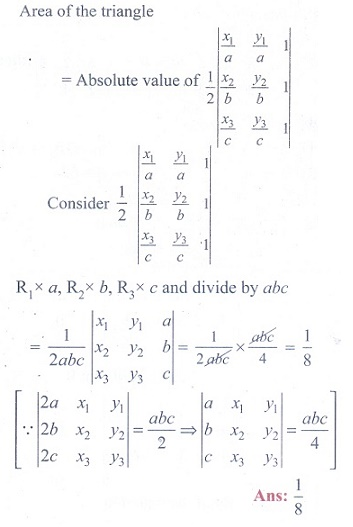Q4. If the square of the matrix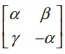is the unit matrix of order 2, then α, β and γ should satisfy the relation.
Explaination / Solution: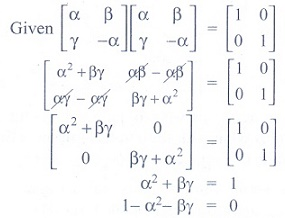Q5. If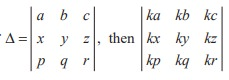is
Explaination / Solution: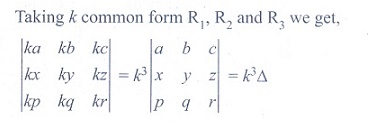Q6. A root of the equation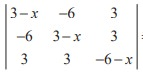= 0 is
Explaination / Solution: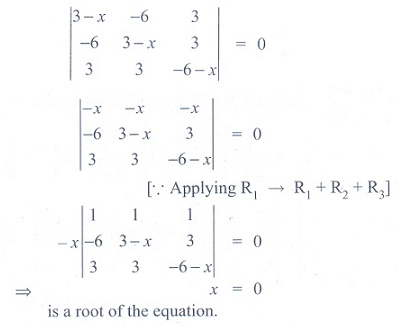Q7. The value of the determinant of A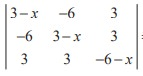is
Explaination / Solution: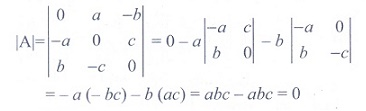Q8. If  x1 , x2 , x3 as well as y1 , y 2 , y3 are in geometric progression with the same common ratio, then the points (x1 , y1 ), (x2 , y2 ), (x3 , y3) are
Explaination / Solution: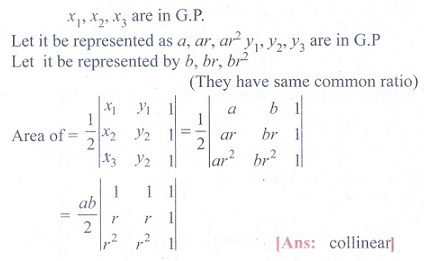Q9. If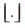denotes the greatest integer less than or equal to the real number under consideration and  −1 ≤ x < 0, 0 ≤ y < 1, 1 ≤ z < 2 , then the value of the determinant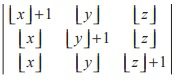is
Explaination / Solution: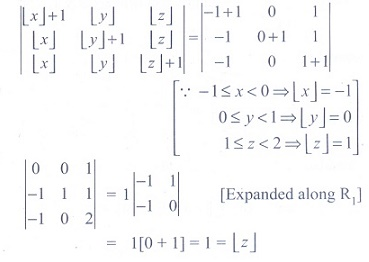Q10. If a ≠ b, b, c satisfy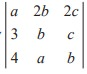= 0, then abc =
Explaination / Solution: